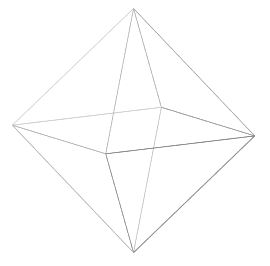Contents

# Contents

## Idea(image source)

One of the Platonic solids

$\,$

$\,$

$\,$

Dynkin diagram/
Dynkin quiver
Platonic solidfinite subgroups of SO(3)finite subgroups of SU(2)simple Lie group
$A_{n \geq 1}$cyclic group
$\mathbb{Z}_{n+1}$
cyclic group
$\mathbb{Z}_{n+1}$
special unitary group
$SU(n+1)$
D4Klein four-group
$D_4 \simeq \mathbb{Z}_2 \times \mathbb{Z}_2$
quaternion group
$2 D_4 \simeq$ Q8
SO(8)
$D_{n \geq 4}$dihedron,
hosohedron
dihedral group
$D_{2(n-2)}$
binary dihedral group
$2 D_{2(n-2)}$
special orthogonal group
$SO(2n)$
$E_6$tetrahedrontetrahedral group
$T$
binary tetrahedral group
$2T$
E6
$E_7$cube,
octahedron
octahedral group
$O$
binary octahedral group
$2O$
E7
$E_8$dodecahedron,
icosahedron
icosahedral group
$I$
binary icosahedral group
$2I$
E8
• Felix Klein, chapter I.7 of Vorlesungen über das Ikosaeder und die Auflösung der Gleichungen vom fünften Grade, 1884, translated as Lectures on the Icosahedron and the Resolution of Equations of Degree Five by George Morrice 1888, online version

• Wikipedia, Octahedron